# Olympiad Test: Pattern - 1

Test Description

## 15 Questions MCQ Test Science Olympiad Class 8 | Olympiad Test: Pattern - 1

Olympiad Test: Pattern - 1 for Class 8 2023 is part of Science Olympiad Class 8 preparation. The Olympiad Test: Pattern - 1 questions and answers have been prepared according to the Class 8 exam syllabus.The Olympiad Test: Pattern - 1 MCQs are made for Class 8 2023 Exam. Find important definitions, questions, notes, meanings, examples, exercises, MCQs and online tests for Olympiad Test: Pattern - 1 below.
 1 Crore+ students have signed up on EduRev. Have you?
Olympiad Test: Pattern - 1 - Question 1

###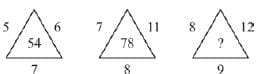Detailed Solution for Olympiad Test: Pattern - 1 - Question 1

(5 + 6 + 7) × 3 = 18 × 3 = 54
(7 + 11 + 8) × 3 = 26 × 3 = 78
∴ ? = (8 + 12 + 9) × 3 = 29 × 3 = 87

Olympiad Test: Pattern - 1 - Question 2

###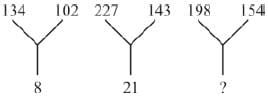Detailed Solution for Olympiad Test: Pattern - 1 - Question 2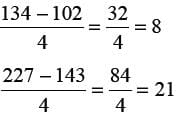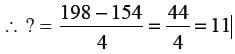Olympiad Test: Pattern - 1 - Question 3

###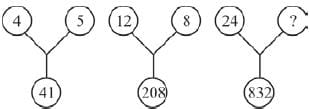Detailed Solution for Olympiad Test: Pattern - 1 - Question 3

42 + 52 = 16 + 25 = 41
122 + 82 = 144 + 64 = 208
⇒ 242 + x2 = 832
⇒ x2 = 832 – 576 = 256
x=16

Olympiad Test: Pattern - 1 - Question 4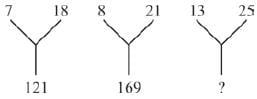Detailed Solution for Olympiad Test: Pattern - 1 - Question 4

(18 – 7)2 = 112 = 121
(21 – 8)2 = 132 = 169
∴ ? = (25 – 13)2 = 122 = 144

Olympiad Test: Pattern - 1 - Question 5Detailed Solution for Olympiad Test: Pattern - 1 - Question 5

(25) 2 – (18) 2 = 625 – 324 = 301
(27) 2 – (16) 2 = 729 - 256 = 473
∴ ? = (23) 2 – (9) 2 = 529 – 81 = 448

Olympiad Test: Pattern - 1 - Question 6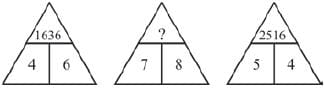Detailed Solution for Olympiad Test: Pattern - 1 - Question 6

42 62 = 1636
52 42 = 2516
∴ ? = 72 82 = 4964;

Olympiad Test: Pattern - 1 - Question 7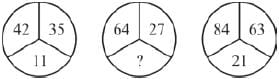Detailed Solution for Olympiad Test: Pattern - 1 - Question 7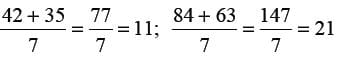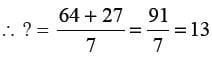Olympiad Test: Pattern - 1 - Question 8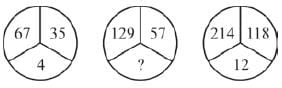Detailed Solution for Olympiad Test: Pattern - 1 - Question 8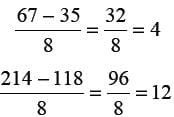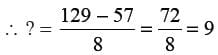Olympiad Test: Pattern - 1 - Question 9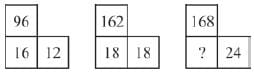Detailed Solution for Olympiad Test: Pattern - 1 - Question 9

(96 ÷ 16) × 2 = 6 × 2 = 12
(162 ÷ 18) × 2 = 9 × 2 = 18
and (168 ÷ x) × 2 = 24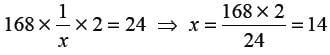Olympiad Test: Pattern - 1 - Question 10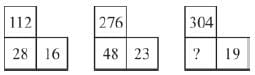Detailed Solution for Olympiad Test: Pattern - 1 - Question 10

(112 ÷ 16) × 4 = 7 × 4 = 28
(276 ÷ 23) × 4 = 12 × 4 = 48
∴ ? = (304 ÷ 19) × 4 = 16 × 4 = 64

Olympiad Test: Pattern - 1 - Question 11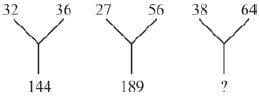Detailed Solution for Olympiad Test: Pattern - 1 - Question 11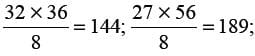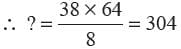Olympiad Test: Pattern - 1 - Question 12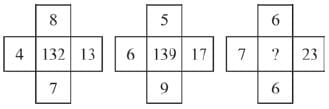Detailed Solution for Olympiad Test: Pattern - 1 - Question 12

(7 × 4) + (8 × 13) = 28 + 104 = 132
(9 × 6) + (5 × 17) = 54 + 85 = 139
∴ ? = (6 × 7) + (6 × 23) = 42 + 138 = 180

Olympiad Test: Pattern - 1 - Question 13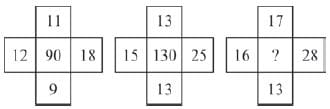Detailed Solution for Olympiad Test: Pattern - 1 - Question 13

(18 × 11) – (9 × 12) = 198 – 108 = 90
(25 × 13) – (15 × 13) = 325 – 195 = 130
∴ ? = (28 × 17) - (16 × 13) = 476 – 208 = 268

Olympiad Test: Pattern - 1 - Question 14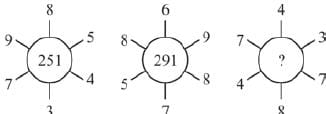Detailed Solution for Olympiad Test: Pattern - 1 - Question 14

985 – 734 = 251; 869 – 578 = 291
∴ ? = 743 – 487 = 256

Olympiad Test: Pattern - 1 - Question 15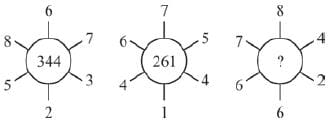Detailed Solution for Olympiad Test: Pattern - 1 - Question 15

867 – 523 = 344; 675 – 414 = 261;
∴ ? = 784 – 662 = 122